DraftKings Championship Odds
+190
1.9 to 1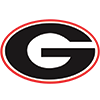Georgia
24.8% implied probability

+200
2 to 1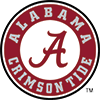Alabama
24.0% implied probability

+240
2.4 to 1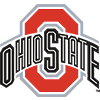Ohio State
21.2% implied probability

+1500
15 to 1Clemson
4.5% implied probability

+1600
16 to 1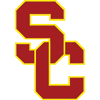USC
4.2% implied probability

+2200
22 to 1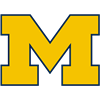Michigan
3.1% implied probability

+5000
50 to 1Utah
1.4% implied probability

+6000
60 to 1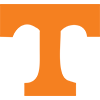Tennessee
1.2% implied probability

+7000
70 to 1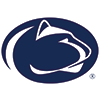Penn State
1.0% implied probability

+10000
100 to 1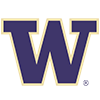Washington
0.7% implied probability

+10000
100 to 1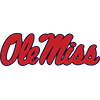Mississippi
0.7% implied probability

+10000
100 to 1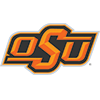Oklahoma State
0.7% implied probability

+10000
100 to 1North Carolina State
0.7% implied probability

+10000
100 to 1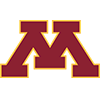Minnesota
0.7% implied probability

+10000
100 to 1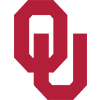Oklahoma
0.7% implied probability

+12000
120 to 1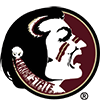Florida State
0.6% implied probability

+12000
120 to 1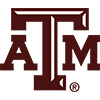Texas A&M
0.6% implied probability

+12000
120 to 1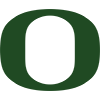Oregon
0.6% implied probability

+15000
150 to 1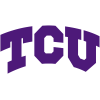TCU
0.5% implied probability

+15000
150 to 1Baylor
0.5% implied probability

+15000
150 to 1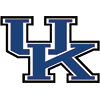Kentucky
0.5% implied probability

+15000
150 to 1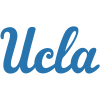UCLA
0.5% implied probability

+15000
150 to 1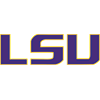LSU
0.5% implied probability

+20000
200 to 1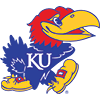Kansas
0.4% implied probability

+20000
200 to 1Kansas State
0.4% implied probability

+20000
200 to 1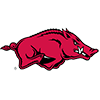Arkansas
0.4% implied probability

+30000
300 to 1Wake Forest
0.2% implied probability

+30000
300 to 1Syracuse
0.2% implied probability

+30000
300 to 1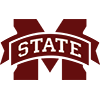Mississippi State
0.2% implied probability

+30000
300 to 1Pittsburgh
0.2% implied probability

+30000
300 to 1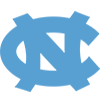North Carolina
0.2% implied probability

+40000
400 to 1Wisconsin
0.2% implied probability

+40000
400 to 1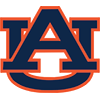Auburn
0.2% implied probability

+40000
400 to 1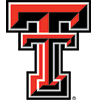Texas Tech
0.2% implied probability

+40000
400 to 1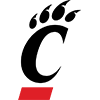Cincinnati
0.2% implied probability

+40000
400 to 1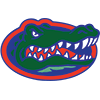Florida
0.2% implied probability

+40000
400 to 1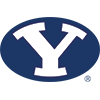BYU
0.2% implied probability

+40000
400 to 1Oregon State
0.2% implied probability

+50000
500 to 1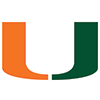Miami
0.1% implied probability

+50000
500 to 1Washington State
0.1% implied probability

+50000
500 to 1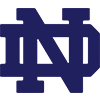Notre Dame
0.1% implied probability

+50000
500 to 1Iowa
0.1% implied probability

+50000
500 to 1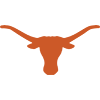Texas
0.1% implied probability

+50000
500 to 1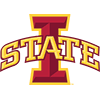Iowa State
0.1% implied probability

+50000
500 to 1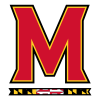Maryland
0.1% implied probability

+80000
800 to 1Central Florida
0.1% implied probability

+80000
800 to 1Coastal Carolina
0.1% implied probability

+100000
1000 to 1UNLV
0.1% implied probability

+100000
1000 to 1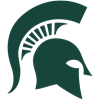Michigan State
0.1% implied probability

+100000
1000 to 1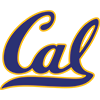California
0.1% implied probability

+100000
1000 to 1Duke
0.1% implied probability

+100000
1000 to 1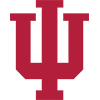Indiana
0.1% implied probability

+100000
1000 to 1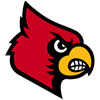Louisville
0.1% implied probability

+100000
1000 to 1Memphis
0.1% implied probability

+100000
1000 to 1Missouri
0.1% implied probability

+100000
1000 to 1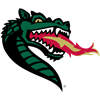UAB
0.1% implied probability

+100000
1000 to 1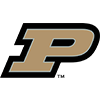Purdue
0.1% implied probability

+100000
1000 to 1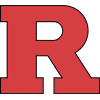Rutgers
0.1% implied probability

+100000
1000 to 1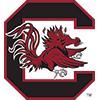South Carolina
0.1% implied probability

+100000
1000 to 1Western Kentucky
0.1% implied probability

+100000
1000 to 1West Virginia
0.1% implied probability

+100000
1000 to 1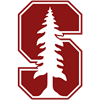Stanford
0.1% implied probability

+100000
1000 to 1Tulane
0.1% implied probability

+100000
1000 to 1Virginia Tech
0.1% implied probability

+100000
1000 to 1Virginia
0.1% implied probability

+100000
1000 to 1Air Force
0.1% implied probability

+200000
2000 to 1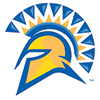San Jose State
0.0% implied probability